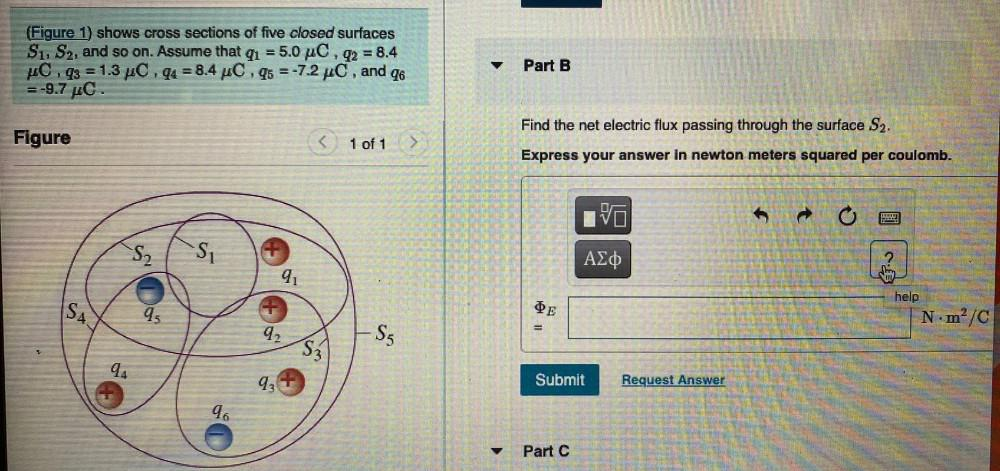Question:

# = 1 (Figure 1) shows cross sections of five closed surfaces S1, S2, and so on. Assume that qı = 5.0 C , q2 = 8.4 C.q3 = 1.3 C.qu= 1 (Figure 1) shows cross sections of five closed surfaces S1, S2, and so on. Assume that qı = 5.0 C , q2 = 8.4 C.q3 = 1.3 C.qu = 8.4 C.q5 = -7.2 C, and 76 = -9.7 C. Part B 3 Figure < 1 of 1 Find the net electric flux passing through the surface S2. Express your answer in newton meters squared per coulomb. -S2 to AEO 91 SA 95 Il lei E help N·mº/C 92 S5 S3 94 93 Submit Request Answer 96 Part C